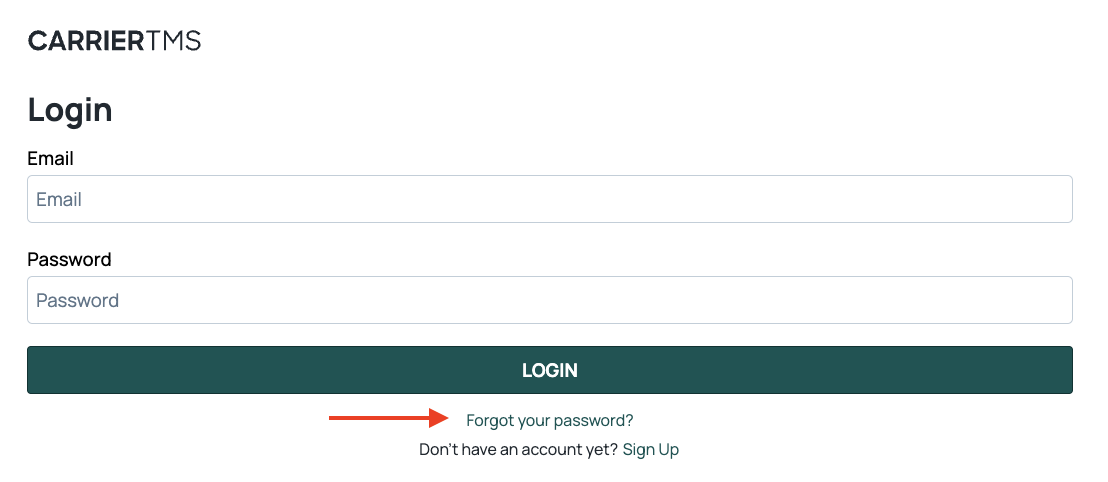• Updated

Important: If the account manager no longer has access to the email address registered to their Carrier TMS user account, contact our support team for further assistance.

1. On your computer or tablet, open a web browser and go to the Carrier TMS Login page.3. Enter the email address that you used to register your account and click Reset My Password.

You will be redirected back to the Carrier TMS Login page. Enter your new credentials to access your account.

0 out of 0 found this helpful

<% if (previousArticle || nextArticle) { %>
<% if (previousArticle) { %>
<% if (previousTitle) { %>

#### <%= previousTitle %>

<% } %> <%= previousArticle.title %>
<% } %> <% if (nextArticle) { %>
<% if (nextTitle) { %>

#### <%= nextTitle %>

<% } %> <%= nextArticle.title %>
<% } %>
<% } %>
<% if (allItems.length > 1) { %>

<% } %>
<% var getColumnClasses = function(numberColumns) { var classNames = 'col-12'; if (numberColumns >= 2) classNames += ' md:col-6'; if (numberColumns >= 3) classNames += ' lg:col-4'; if (numberColumns >= 4) classNames += ' xl:col-3'; return classNames; } %>

##<%= heading %>

<% } %>
<% blocks.forEach(function(block, index) { %>
• <% if (imageHeight) { %><% } %> <% if (block.name) { %>

## <%= block.name %>

<% } %> <% if (block.description) { %>

<%= block.description %>

<% } %>
• <% }) %>

##Featured articles

<% if (articles.length) { %>
<% articles.forEach(function(article) { %>
<%= Util.getExcerpt(article.body, 0) %>
<% }); %>
<% } else { %>

empty

<% } %>
<% var getColumnClasses = function(numberColumns) { var classNames = 'col-12 mb-4'; if (numberColumns >= 2) classNames += ' md:col-6'; if (numberColumns >= 3) classNames += ' lg:col-4'; if (numberColumns >= 4) classNames += ' xl:col-3'; return classNames; } %>

##<%= heading %>

<% } %>
<% blocks.forEach(function(block, index) { %>
• <% if (imageHeight) { %><% } %>
<% if (block.name) { %>

### <% if (block.html_url) { %> <%= block.name %> <% } else { %> <%= block.name %> <% } %>

<% } %> <% if (block.description) { %>

<%= block.description %>

<% } %>
• <% }) %>

Didn't find what you were looking for?

<% if (Theme.callToActionButtons && Theme.callToActionButtons.community.length) { %> <% Theme.callToActionButtons.community.forEach(function(button) { %> <% }) %> <% } %>
<% categories.forEach(function(category, index) { %>
• <% }); %>
<% var maxSections = 5 %>
<% if (sections.length) { %>
<% sections.forEach(function(section) { %>
• <%= partial('partial-article-list-sections', { id: 'section-' + section.id, parentId: '#' + id, sections: section.sections, activeCategoryId: activeCategoryId, activeSectionId: activeSectionId, activeArticleId: activeArticleId, partial: partial }) %> <% if (section.articles.length) { %> <% } %>
• <% }); %>
<% } %>
<% if (sections.length) { %>
<% sections.slice(0, maxSections).forEach(function(section) { %>
• <%= section.name %> <%= partial('partial-section-list-sections', { parent: section, sections: section.sections, maxSections: maxSections, partial: partial }) %>
• <% }); %> <% if (sections.length > maxSections) { %>
• See more
• <% } %>
<% } %>